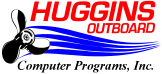2/2019 price files are available.

```02/2019 = 337,761 parts.
01/2019 = 336,836 parts.
12/2018 = 336,737 parts.
11/2018 = 336,134 parts.
10/2018 = 334,698 parts.
09/2018 = 334,601 parts.
08/2018 = 334,142 parts.
07/2018 = 333,445 parts.
06/2018 = 333,058 parts.
05/2018 = 332,328 parts.
04/2018 = 331,575 parts.
03/2018 = 331,348 parts.
02/2018 = 331,185 parts.
01/2018 = 330,949 parts.
```

```For questions about this web page, please contact:
Huggins Outboard         (919) 787-1836
Attn: Robert Huggins     (919) 787-1890 fax
7524 Tanglewild Drive    robert@hugginsweb.com
Raleigh, NC 27613
http://www.hug911.com/suzwin/default.html
```